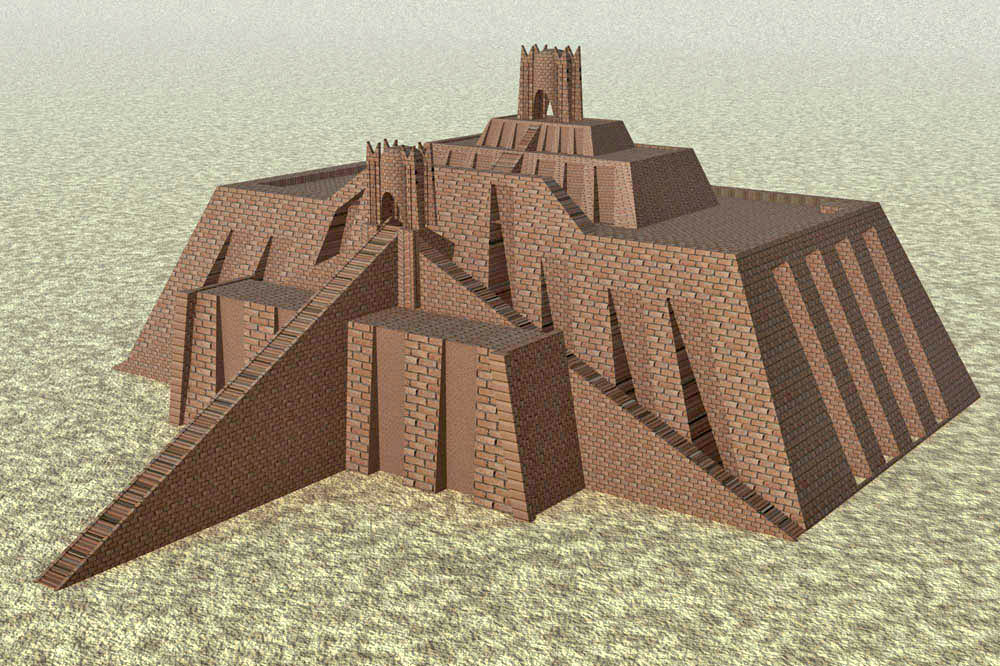# Sullivan Library at WDMS: Grade 6 Research Guides

## Ancient Civilizations^^^^^^^^^^^^^^^^^^^^^^^^^^^^^^^^^^^^^^^^^^^^^^^^^^^^^^^^^^^^^^^^^^^^^^^^^^^^^^^^^^^^^^^^^^^^^^^^^^^^^^^^^^^^^^^^^^^^^^^^^^^^^^^^^^^^^^^^^^^^^^^^^^^^^^^Website Evaluation - Just CART it! A successful researcher always evaluates information, especially from the Internet.  Diamond students use the CART method.

## Real or Fake?

What do you think? Would you trust the information on these websites?

## Website Evaluation ActivityCART  Lesson

Students will work in pairs to
evaluate 2 different websites
using the CART method.

Be prepared to explain why one website is a better resource than the other.

Each pair will need:
1 CART checklist & 1 ChromebookGROUP A - Queen Nefertiti
website #1
website #2GROUP B - Ziggurat